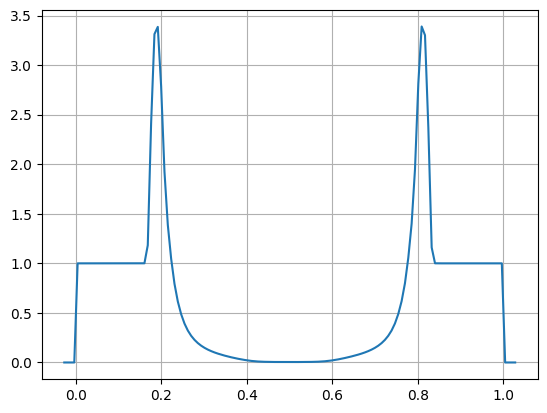# Working with output

## Utilities

Several simply utilities exist to operate on output files

• `compare.py:` this script takes two plot files and compares them zone-by-zone and reports the differences. This is useful for testing, to see if code changes affect the solution. Many problems have stored benchmarks in their solver’s tests directory. For example, to compare the current results for the incompressible shear problem to the stored benchmark, we would do:

```./compare.py shear_128_0216.pyro incompressible/tests/shear_128_0216.pyro
```

Differences on the order of machine precision may arise because of optimizations and compiler differences across platforms. Students should familiarize themselves with the details of how computers store numbers (floating point). An excellent read is What every computer scientist should know about floating-point arithmetic by D. Goldberg.

• `plot.py`: this script uses the solver’s `dovis()` routine to plot an output file. For example, to plot the data in the file `shear_128_0216.pyro` from the incompressible shear problem, you would do:

```./plot.py -o image.png shear_128_0216.pyro
```

where the `-o` option allows you to specify the output file name.

pyro output data can be read using the `util.io_pyro.read` method. The following sequence (done in a python session) reads in stored data (from the compressible Sedov problem) and plots data falling on a line in the x direction through the y-center of the domain (note: this will include the ghost cells).
```import matplotlib.pyplot as plt